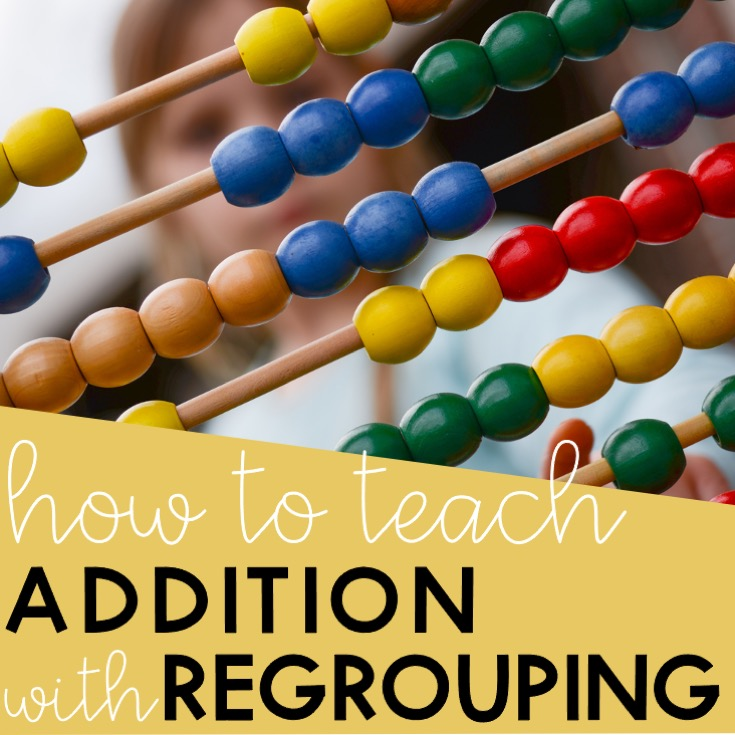Addition with regrouping using the standard algorithm is one of the common core skills required for primary or elementary students. Doing column addition gets tricky when students are asked to regroup or ‘carry’ a number to the next column. This gets really confusing for students especially when they lack place value understanding.

Here are two ways on how you can teach addition with regrouping to your students. Help them transition their thinking from visual to abstract by doing these two things.

## Using place value models

Manipulatives are helpful for students in visualizing any concept. When teaching addition with regrouping, it is helpful to use visual models such as base ten blocks or place value models. Even a quick picture of these models will help your students understand how regrouping works.

Let’s say you’re adding 327 + 189. Represent the addends by using place value models. Before adding, remind your students of the value of each block or model and what they represent.

To add using place value models or base ten blocks, first, align the numbers and models in columns.

Then, have them look at the ones column and ask: “How many ones are there?” There are 16 ones.

Since there are 16 ones, have them think of other ways to represent the number. Have them think and wonder of another way to represent 16 ones using the model. Your goal is to have them think of 16 ones as 1 tens and 6 ones.

Now that they know that 16 ones is 1 tens and 6 ones, show the regrouping by encircling the 10 ones. Cross out the 10 ones and move it to the tens column by adding 1 tens. Keep the 6 ones in the ones column.

Next, continue adding the tens column. Have them think of the number of tens there are and ask, “What is another way to represent 11 tens?” It is key to remind them that 10 tens is the same as 1 hundreds. Help them think of 11 tens as 1 hundreds and 1 tens.

Again, show the regrouping by encircling the 10 tens and representing it with a 1 hundred and 1 ten. Keep the 1 ten under the tens column and move the 1 hundred model in the hundreds column.

Now, add the hundreds. Let them count the number of hundreds they see including the regrouped hundreds.

Transition using place value models to the standard algorithm by writing the numbers in columns too as represented by these models.

## Using a place-value chart

Place-value charts help students figure out where a digit is in the number. By knowing where the digit is, will help your students know the value of each digit. Using a place value chart when teaching addition with regrouping is a great tool.

Let’s say you are adding 438 + 275. Show a place value chart up to the hundreds place. Help students place the digits in each addend on the place value chart. Emphasize that by placing each digit in the place value chart, the addends will be aligned according to their place value.

Start adding the addends from the ones place. Read it as 8 ones + 5 ones = 13 ones. Help students realize that 13 ones is the same as 1 tens and 3 ones. Thus, you need to ‘carry over’ or regroup the 1 tens to the tens place.

Continue adding the tens place making sure to add the regrouped 1 tens. While adding, read the digits as 1 tens + 3 tens + 7 tens = 11 tens. Again, help your students think of 11 tens as 1 hundreds and 1 tens.

Finish the addition by adding the digits in the hundreds place. Remind students to include the regrouped hundreds.

By starting off with models and using place-value charts, you can help students understand how regrouping in addition works. Remember that you can always scale back to two-digits or even to one-digit addition when you see that your students are having a difficult time.